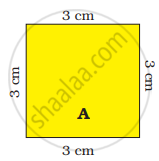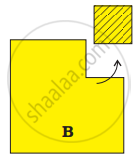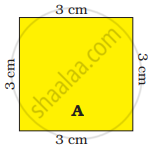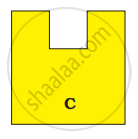# A square has a boundary of 12 cm. From the corner of this square, a small square of side 1 cm is cut off. Will the boundary of B be less or more? Find its length. If you cut a 1 c - Mathematics

A square has a boundary of 12 cm.

1. From the corner of this square, a small square of side 1 cm is cut off. Will the boundary of B be less or more? Find its length.
2. If you cut a 1 cm square to get shape C, what will be the length of the boundary of C?Square New shape

#### Solution

1. The boundary of shape ‘B’ is equal to the boundary of shape ‘C’
The boundary of ‘B’ = 12 cm.
2. The boundary of shape ‘C’ will be equals to 14 cm.
Concept: Fields and Fences
Is there an error in this question or solution?

#### APPEARS IN

NCERT Mathematics - Math - Magic Class 4 CBSE
Chapter 13 Fields and Fences
Q 1. | Page 155